# Write a Program to Find Sum of Digits in Java

One of the common programming practice question thrown to beginners is to write a program to calculate the sum of digits in an integral number. For example, if the input is 123456 then output or sum of the digit is (1+2+3+4+5+6) = 21. An additional condition is you can not use any third party or library method to solve this problem. This program is not as simple as it looks and that's why it's a good exercise, you must know some basic programming techniques e.g. loops, operators, and logic formation to solve this problem. Let's see how we can solve this problem using Java programming language. In order to calculate the sum of digits, we must get digits as numbers. So your first challenge is how do you get the digits as numbers?  How do we extract 6 out of 123456?

If you have done exercises like palindrome check or reversing number, then you should know that there is very old technique of getting last digit from a number by using modulus operator. If we do 123456%10 then we will get 6, which is last digit. In order to get all digits we can use a loop, something like while loop.

Now our next challenge is how do we reduce number in each iteration so that our loop will finish as soon as we are done with all digits of number? Now coming from same palindrome problem, you can use technique of dividing number by 10 to get rid of last digit or reduce it by factor of 10.

For example 123456/10 will give you 12345, which is one digit less than original number. So you got your end condition for while loop, check until number is not equal to zero. These two techniques are very important and can be used in variety of problem, so always remember these.

## Java program to find Sum of Digits in Java

Here is our complete Java program to solve this problem. As explained in first paragraph, it does not use any library method instead uses division and modulus operator to calculate sum of digits of a number.

```import java.io.Console;
import java.util.Scanner;
import java.util.concurrent.Semaphore;
import java.util.concurrent.locks.Condition;
import java.util.concurrent.locks.Lock;
import java.util.concurrent.locks.ReentrantLock;

/**
*
* How to find sum of digits in Java
*
* @author Javin Paul
*/
public class SumOfDigits{

public static void main(String args[]) {

Scanner sc = new Scanner(System.in);

System.out.println("Please enter a number to calculate sum of digits");
int number = sc.nextInt();

// Remember number/10 reduces one digit from number
// and number%10 gives you last digit
int sum = 0;
int input = number;
while (input != 0) {
int lastdigit = input % 10;
sum += lastdigit;
input /= 10;
}

System.out.printf("Sum of digits of number %d is %d", number, sum);

// closing Scanner to prevent resource leak
sc.close();

}

}Please enter a number to calculate sum of digits
101
Sum of digits of number 101 is 2

Please enter a number to calculate sum of digits
123
Sum of digits of number 123 is 6```

That's all on how do you calculate sum of digits of a number in Java. It's an interesting exercise and you are welcome to find other solution as well. Try not to see the solution before doing it because if you can come up with logic by your own, you will learn a lot. These kind of programs are good for learning basic programming techniques and developing coding sense. If you are interested, you can find lot of such questions in this blog e.g. checkout this 10 programming questions article.

Further Learning
The Coding Interview Bootcamp: Algorithms + Data Structures
Data Structures and Algorithms: Deep Dive Using Java
Algorithms and Data Structures - Part 1 and 2

#### 19 comments:

1.import java.util.concurrent.Semaphore;
import java.util.concurrent.locks.Condition;
import java.util.concurrent.locks.Lock;
import java.util.concurrent.locks.ReentrantLock;

??

2.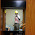Hi there!

I don't think it's necessary for you to use the four classes you've imported from the java.util.concurrent package, because that is more for parallel programming. You got multiple threads trying to access a common element at the same time, but you would use those classes to avoid thread locks. In addition, I can't seem to understand why you need to import java.io.Console, when the System class is already imported.

Anyway, very good article. What do you say you check out my Blogger post? Suggestions are welcome! http://gregorypdesrosiersmontreal.blogspot.com/2014/06/rock-paper-scissors-lizard-spock-java.html

Please let me know!

1.Hello @Anonymous and @Gregory, those imports are not warranted in this program. It seems they are just left from another exercise and some how I forgot to remove them. Anyway, thanks for pointing that.

Regards
Javin

3.for(char c : "123456".toCharArray())
{
sum += (int)(c - '0');
}

4.Gotta love recursion (excuse formatting).

public static int digitalRoot(int x) {
if (x < 10) {
return x;
}
return digitalRoot(x / 10) + digitalRoot(x % 10);
}

5.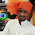may string is : abcd10bh gsi20gdf gsjb30gj.

and i wand output as 10+20+30=60.

in java

6.Can you please show the program using for loop instead of while?

7.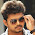i also need the number of digits this must be in thread concept

8.can u plzz help in in finding the number of digits too

1.Try using int count++; inside the while loop :)

9.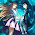can you program this given problem?

"Create a Program that accepts an integer and outputs the sum of all digits..
Example:12345
output=15

10.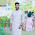class Sum{
public static void main(String[] ar){
int a=12345, sum=0, rem=0;
while(a!=0){
rem=a%10;
a=a/10;
sum=sum+rem;
}

System.out.println(sum);

}

}

11.if u create a program in which both coding & GUI format r visible it will help me more bcs im a beginner

12.how to Get the sum of the numbers that ends with 0. Display the sum in java
That lets user enter 10 numbers...

13.Without loop.. Or use of any function a simple programe.

14.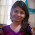can someone plz solve this question..??
Write a java program on-

The sum of the digits of a two-digit number is 15 and the difference between the digits is 3. Write a program to find out the two-digit number.

1.// Write a program to find out the two digit number whose sum of the digits is 15 and the difference between the digits is 3.

class FindNum{
public static void main(String[] args){
int a=0, b=0, sum, diff;
for(a=0;a<10;a++)
{
for(b=0;b<10;b++)
{
if((a+b)==15 && (a-b)==3)
{
System.out.println("Value of a: "+a);
System.out.println("Value of b: "+b);
}
}
}
}
}

15.How from left to right

16.Without using any loop r control statements